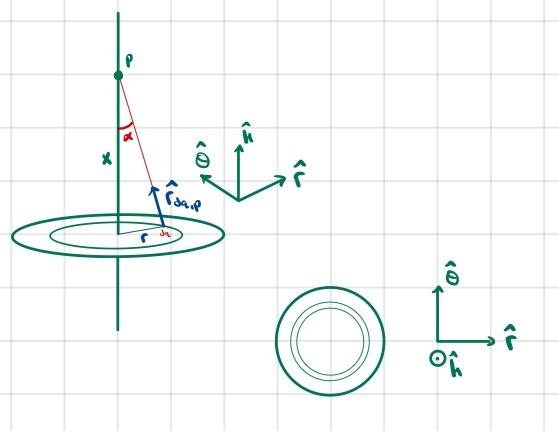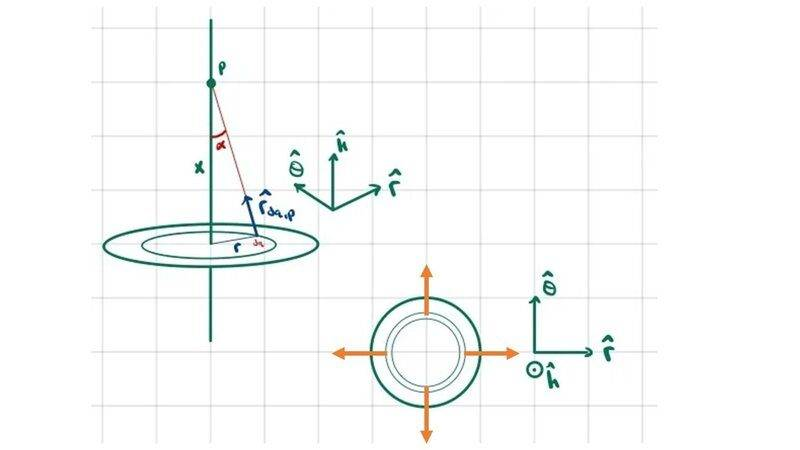# Calculate the electric field due to a charged disk (how to do the integration?)

zenterix
Homework Statement:
A disc of radius ##R## is uniformly charged with total charge ##Q>0##. Determine the direction and magnitude of the electric field at a point P lying a distance ##x>0## from the center of the disc along the axis of symmetry of the disc.
Relevant Equations:
I know how to solve this problem. The electric field vector points in only one direction, so there is really only one integral to do. However, I attempted to do the full integral, expecting the other two directions to cancel out, but my integrals don't seem to work out to the correct values. This is thus a question about integration.

Here I set up the integral for the electric field due to the disk at a point ##P##:

$$d\vec{E}_p=\frac{k_edq}{r_{dq,p}^2}\hat{r}_{dq,p}$$

$$dq=2\pi r dr \frac{Q}{\pi R^2}=\frac{2Qrdr}{R^2}$$

$$\vec{r}_{dq,p}=\vec{r}_{o,p}-\vec{r}_{o,dq}=x\hat{k}-r\hat{r}$$

$$r_{dq,p}=\sqrt{x^2+r^2}$$

$$\implies \vec{E}_p=\frac{2k_eQrdr}{R^2(x^2+r^2)^{3/2})}(x\hat{k}-r\hat{r})$$

$$\vec{E}_p=\frac{2k_eQ}{R^2}\left[\int_0^R \frac{rx}{(x^2+r^2)^{3/2}}dr\hat{k}-\int_0^R \frac{r^2}{(x^2+r^2)^{3/2}}dr\hat{r}\right]$$
I am interested in particular in the second integral, in the ##\hat{r}## direction.

Here is my depiction of the problem:As far as I can tell, due to the symmetry of the problem, this integral should be zero.

$$\int_0^R \frac{r^2}{(x^2+r^2)^{3/2}}dr\hat{r}$$

I don't believe I need to integrate anything inside ##\hat{r}##; it depends on an angle relative to some axis but not on ##r##.

$$r=x\tan{\alpha}$$
$$dr=x \sec^2{\alpha}d\alpha$$

$$(x^2+r^2)^{3/2}=(x^2(1+\frac{r^2}{x^2}))^{3/2}=x^3(1+\tan^2{\alpha})^{3/2}=x^3\sec^3{\alpha}$$

So the integral becomes

$$\int \frac{x^2\tan^2{\alpha} x \sec^2{\alpha}}{x^3\sec^3{\alpha}}d\alpha$$
$$=\int \frac{\tan^2{\alpha}}{\sec{\alpha}}d\alpha$$

$$=\int \frac{\sin^2{\alpha}}{\cos{\alpha}}d\alpha$$

$$=\int \frac{1}{\cos{\alpha}}d\alpha-=\int_0^R \cos{\alpha}d\alpha$$

$$=(\ln|\sec{\alpha}+\tan{\alpha}| - \sin{\alpha})$$

Note that

$$r=x\tan{\alpha}$$
$$\implies \sin{\alpha}=\frac{r}{(x^2+r^2)^{1/2}}$$
$$\implies \cos{\alpha}=\frac{x}{(x^2+r^2)^{1/2}}$$

The integral result in terms of ##r## is then

$$(\ln|\frac{(x^2+r^2)^{1/2}}{x}+\frac{r}{x}|-\frac{r}{(x^2+r^2)^{1/2}}) \big|_0^R$$

Which is not zero.

I don't know if I messed up setting up the integral, or in the integration itself.

•Delta2

ergospherical
The issue with your work is that the unit vector ##\hat{\boldsymbol{r}}## depends on the angle ##\varphi## of the charge element in the ring around the ##x## axis. If you want to evaluate the horizontal component of the electric field explicitly, you'll need to e.g. write it out in Cartesians,\begin{align*}
dE_y = \dfrac{Q}{4\pi^2 \epsilon_0 R^2} \dfrac{r^2}{(x^2 + r^2)^{3/2}} \cos{\varphi} dr d\varphi \\
dE_z = \dfrac{Q}{4\pi^2 \epsilon_0 R^2} \dfrac{r^2}{(x^2 + r^2)^{3/2}} \sin{\varphi} dr d\varphi
\end{align*}and do a double integral over ##r## and ##\varphi##. Since they're separable, and the angle ranges over ##[0,2\pi]##, you can see immediately that both will be zero as expected from the cylindrical symmetry.

•PeroK and zenterix
Gold Member
I would get potential which is scalar, easier to handle than vector
$$\phi(x)=k_e \frac{Q}{\pi R^2}\int_0^R \frac{2\pi r dr}{\sqrt{x^2+r^2}}$$
Then
$$E=-\nabla \phi$$

•Delta2, PhDeezNutz and zenterix
zenterix
The issue with your work is that the unit vector ##\hat{\boldsymbol{r}}## depends on the angle ##\varphi## of the charge element in the ring around the ##x## axis. If you want to evaluate the horizontal component of the electric field explicitly, you'll need to e.g. write it out in Cartesians,\begin{align*}
dE_y = \dfrac{Q}{4\pi^2 \epsilon_0 R^2} \dfrac{r^2}{(x^2 + r^2)^{3/2}} \cos{\varphi} dr d\varphi \\
dE_z = \dfrac{Q}{4\pi^2 \epsilon_0 R^2} \dfrac{r^2}{(x^2 + r^2)^{3/2}} \sin{\varphi} dr d\varphi
\end{align*}and do a double integral over ##r## and ##\varphi##. Since they're separable, and the angle ranges over ##[0,2\pi]##, you can see immediately that both will be zero as expected from the cylindrical symmetry.
I thought I didn't have to worry about ##\varphi## because I wrote the integral in terms of infinitesimal rings. ##dq## is the charge on an infinitesimal ring When I integrate only over ##r##, aren't I summing up all the rings already?

I can see the intuition that I do need to somehow integrate over something that will cancel out, and the ##\hat{r}## vectors all cancel each other out when we consider ##\varphi## from 0 to ##2\pi##, but I can't understand the intuition based on the sum of the rings.

ergospherical
If you want to find the vertical component of the electric field, then you can exploit the cylindrical symmetry by using ring-shaped charge elements of area ##2\pi r dr##. (The factor of ##2\pi## is equivalent to what you'd get if you wrote the double integral over the measure ##rdr d\varphi##, separated the ##r## and ##\varphi## integrals and put ##\displaystyle{\int_{0}^{2\pi}} d\varphi = 2\pi##.)

If you're considering the horizontal field, however, points at different angles around the ring produce contributions to the field at ##P## with different projections onto the ##y## and ##z## axes (depending on the angle). Different points on the ring are no longer equivalent, so you have no choice but to break it up into even smaller bits of area, ##rdrd\varphi##.

•PeroK and zenterix
Gold Member
They seem to cancel in different ##\theta##.Staff Emeritus
Homework Helper
I thought I didn't have to worry about ##\varphi## because I wrote the integral in terms of infinitesimal rings. ##dq## is the charge on an infinitesimal ring When I integrate only over ##r##, aren't I summing up all the rings already?
The field due to the ring points along the z-axis, so you've already integrated out x- and y- components. Try doing the full integral for the field of a ring of charge. That's where you'll see the cancellation occurs.

zenterix
They definitely do cancel, that is clear by simple inspection.

And here's what I came up with.

There was a step where I wrote

$$\vec{r}_{dq,p}=\vec{r}_{o,p}-\vec{r}_{o,dq}$$

The issue is I wrote an expression for ##\vec{r}_{o,dq}##, the vector from the origin to ##dq##, but ##dq## is actually a ring. There isn't a single vector that points to it.

I need to actually consider a small ##dq## on the ring.

##dq_{ring}=\frac{2rQdr}{R^2}##

But now, I calculate a small charge on the ring

##dq=\frac{dq_{ring}}{2\pi r}=\frac{rQdrd\varphi}{\pi}##

Now the vector ##\vec{r}_{o,dq}## actually points to this small charge on the ring, which has a ##d\varphi## term in it.

$$d\vec{E}_p=\frac{k_erQdrd\varphi}{\pi(x^2+r^2)^{3/2}}(x\hat{k}-r\hat{r})$$

Now when we sub in ##\hat{r}=\cos{\varphi}\hat{i}+\sin{\varphi}\hat{j}##. The resulting integrals in ##\hat{i}## and ##\hat{j}## are 0 as expected.

Also, in the ##\hat{k}## direction we get the same result as before as well:

$$\frac{k_eQ}{R^2\pi}\int_0^{2\pi} \int_0^R \frac{rx}{(x^2+r^2)^{3/2}}drd\varphi$$
$$=\frac{2k_eQ}{R^2}\left [ \frac{x}{|x|} - \frac{x}{(x^2+r^2)^{3/2}} \right ]$$

Now, when this problem was done in a textbook, the symmetry argument was used to not do the integral in the ##\hat{r}## direction. However, the integral in the ##\hat{k}## direction (if my calculations above are correct) is also a double integral. Yet when using the symmetry argument we use a single integral to calculate the ##\hat{k}## direction electric field.

•